CIM Created Edited

# [CIM/Rebar] I'm curious about the method of Rebar Modeling, such as Tendon Blister, which is used for non-linear reinforcement modeling.

## Question

How to rebar model atypical structures?

For atypical structures, Rebar Modeling is carried out by using the Rebar Group function, Sweep Array function, and Void Member function.

Info

In CIM, general rebar modeling is created by referring to the edge or point of an object, and based on this, a tapered array that can respond to changes in member shape is used.
However, if the shape change of the object and the reinforcing bar is not the same, a different method of Rebar Modeling is required.
This document deals with Rebar Modeling of atypical structures through Blister Model.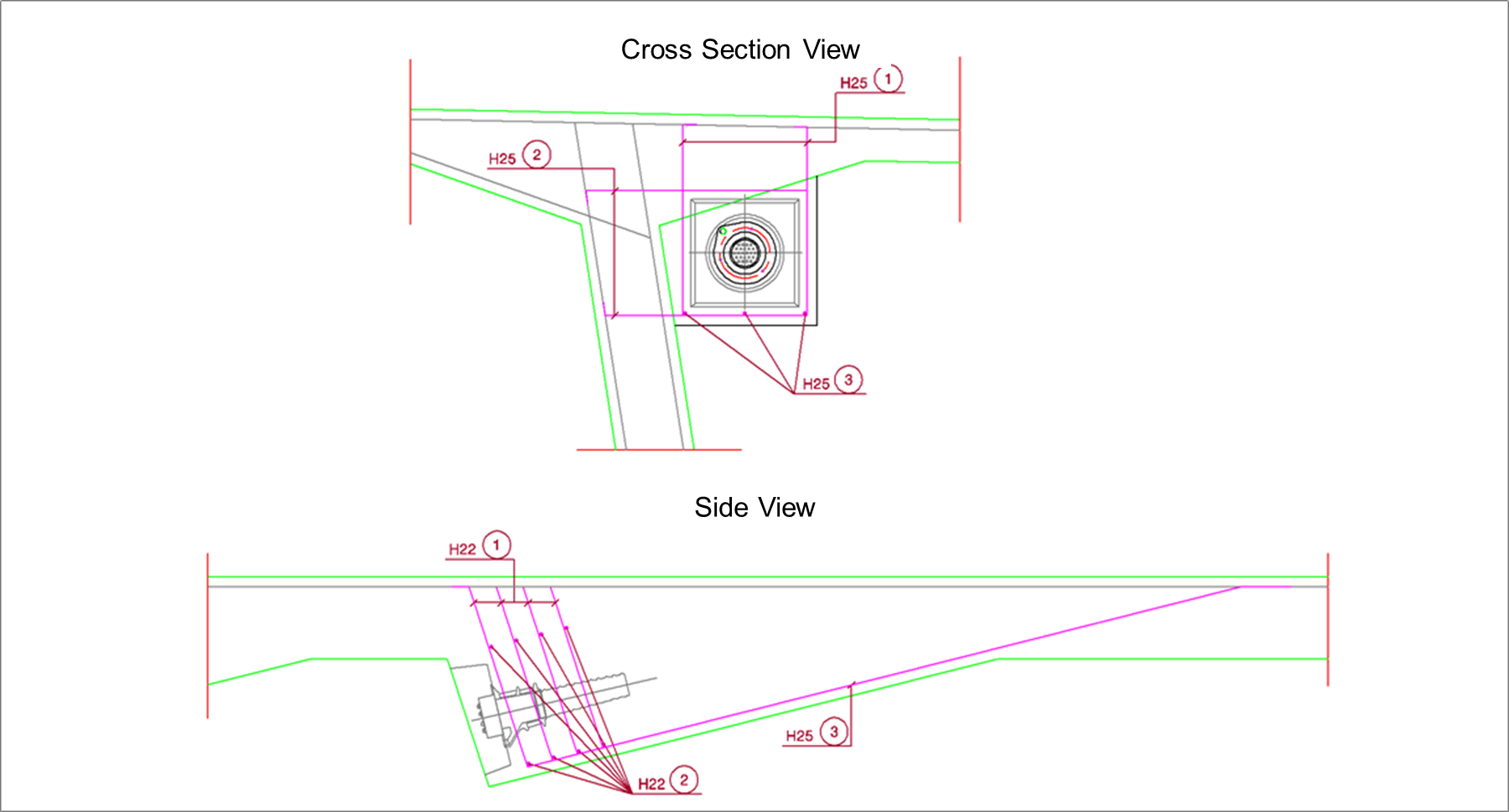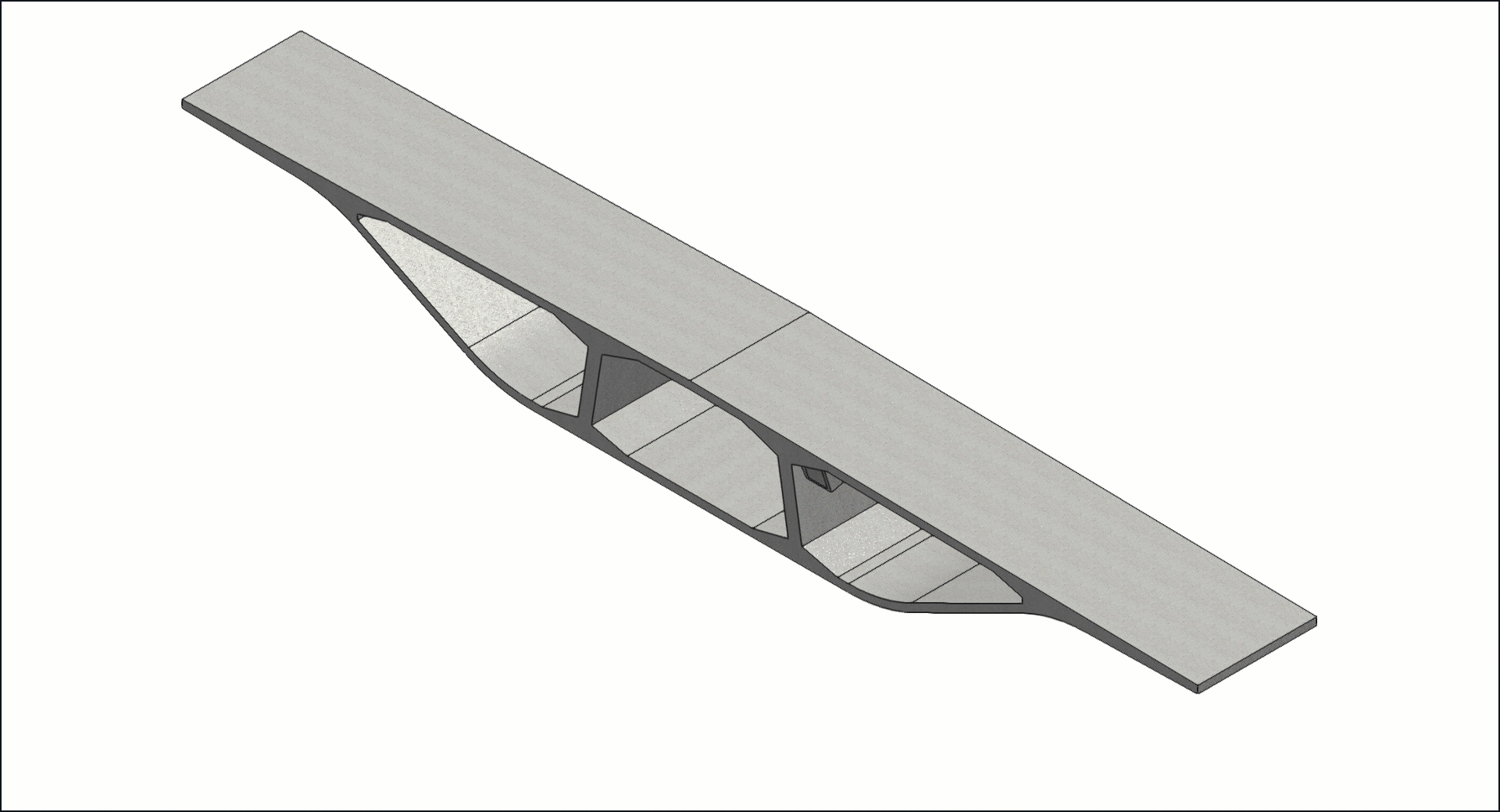Figure. 1 Blister DWG Figure. 2 CIM Blister Model

Figure 1 is a layout diagram of a simplified tendon anchorage for purposes of example. Figure 2 is a shape model of the anchorage created using the drawing.

Using the above model, we will look at three methods of Rebar Modeling of atypical structures.

Case 1.
RModeling using Rebar Group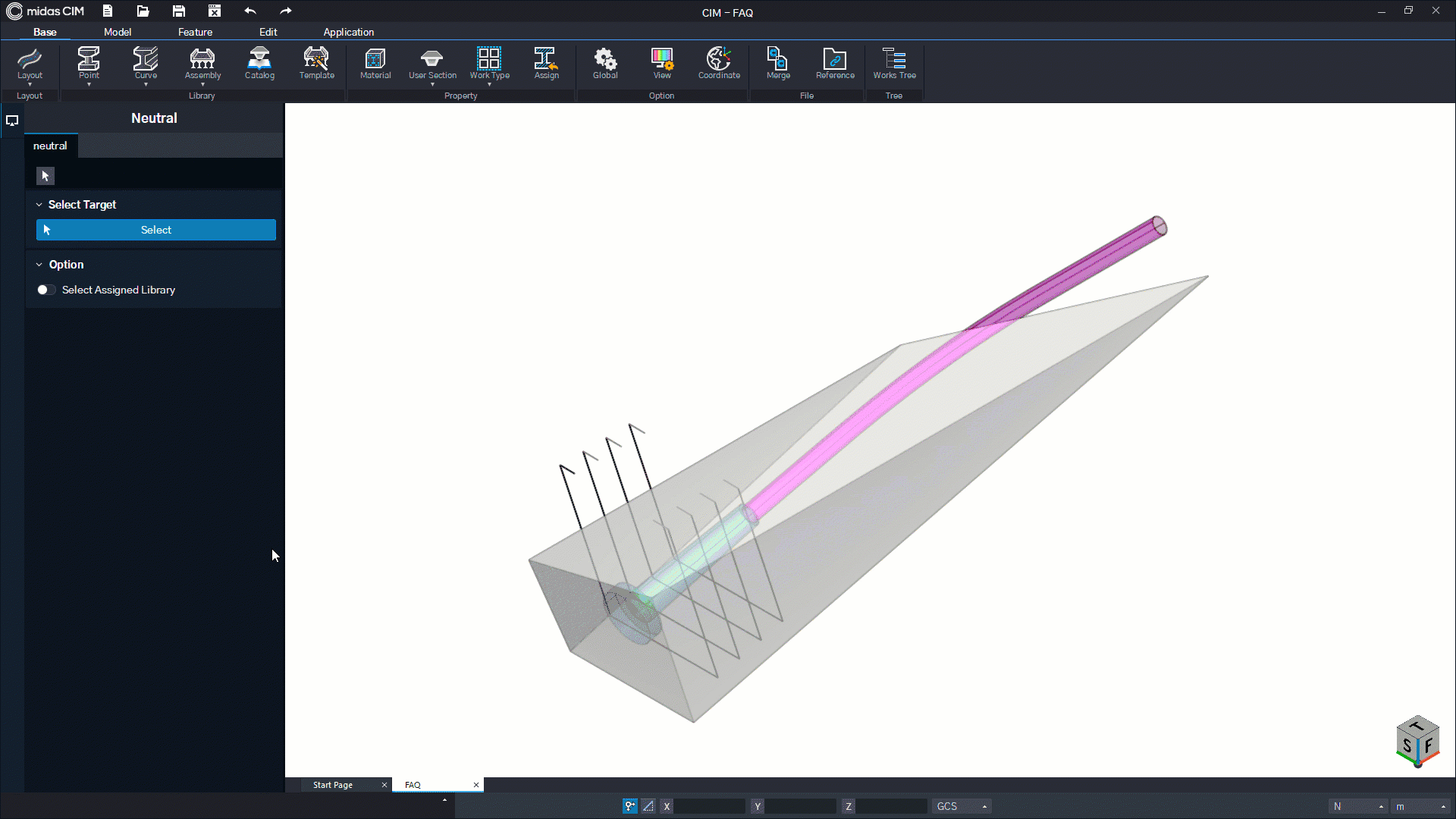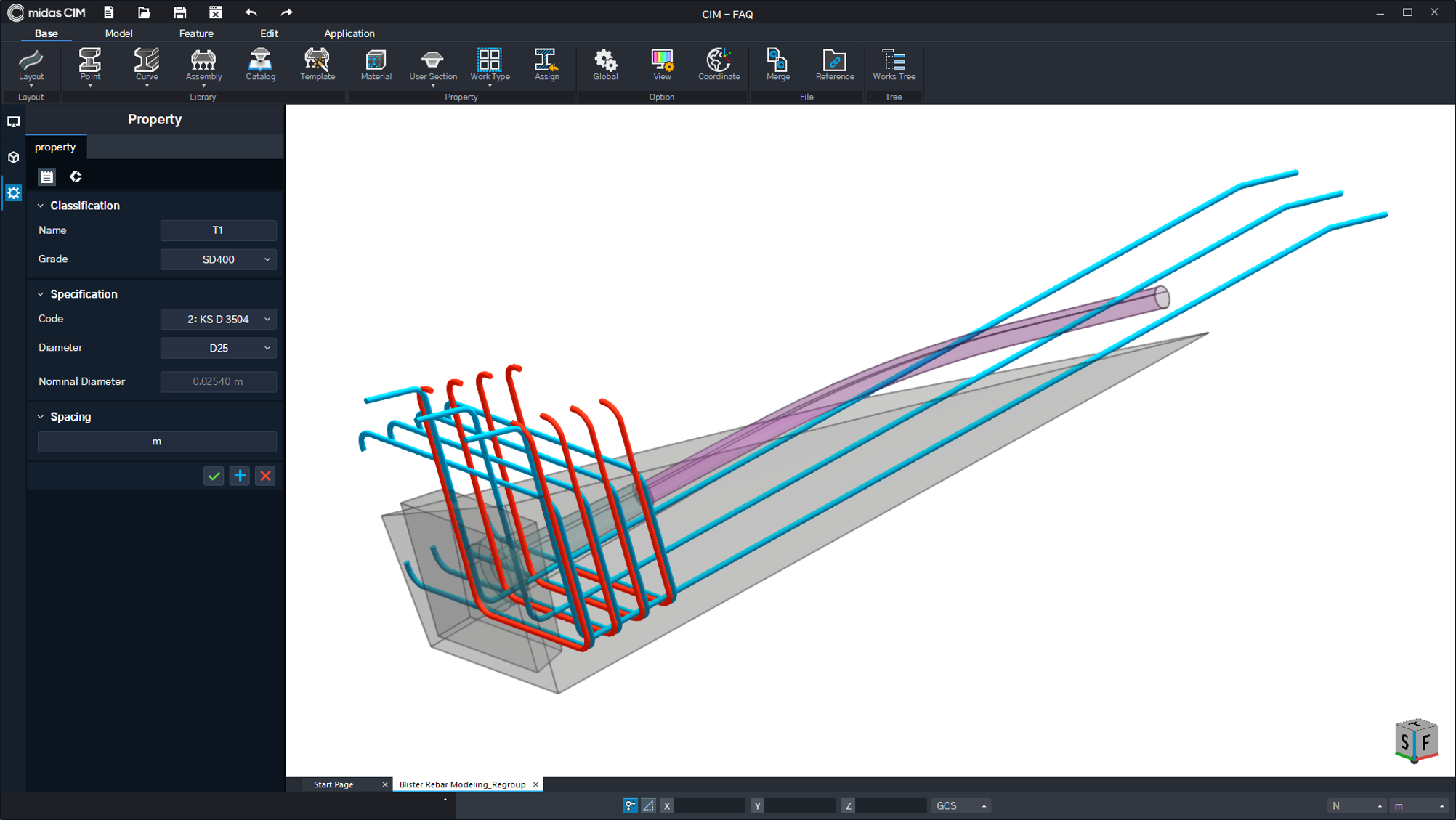Figure. 3 Regroup operation Figure. 4 Modeling result through regroup

Figure 3 shows modeling using the Regroup operation of the Rebar Group function.

Regroup is a function that instantly makes a set of lines sharing a contact point into one rebar.
This function is very convenient because you can work by importing rebar drawn from CAD, etc.

Also, since the rebar is created as an independent object rather than being dependent on the concrete member, it has the advantage of being free in various editing functions.

On the other hand, there may be restrictions such as that it is difficult to modify based on properties because there is no dependency relationship with the concrete member.
(Specifications other than shape information can be modified)

Case 2.
Modeling using Sweep Array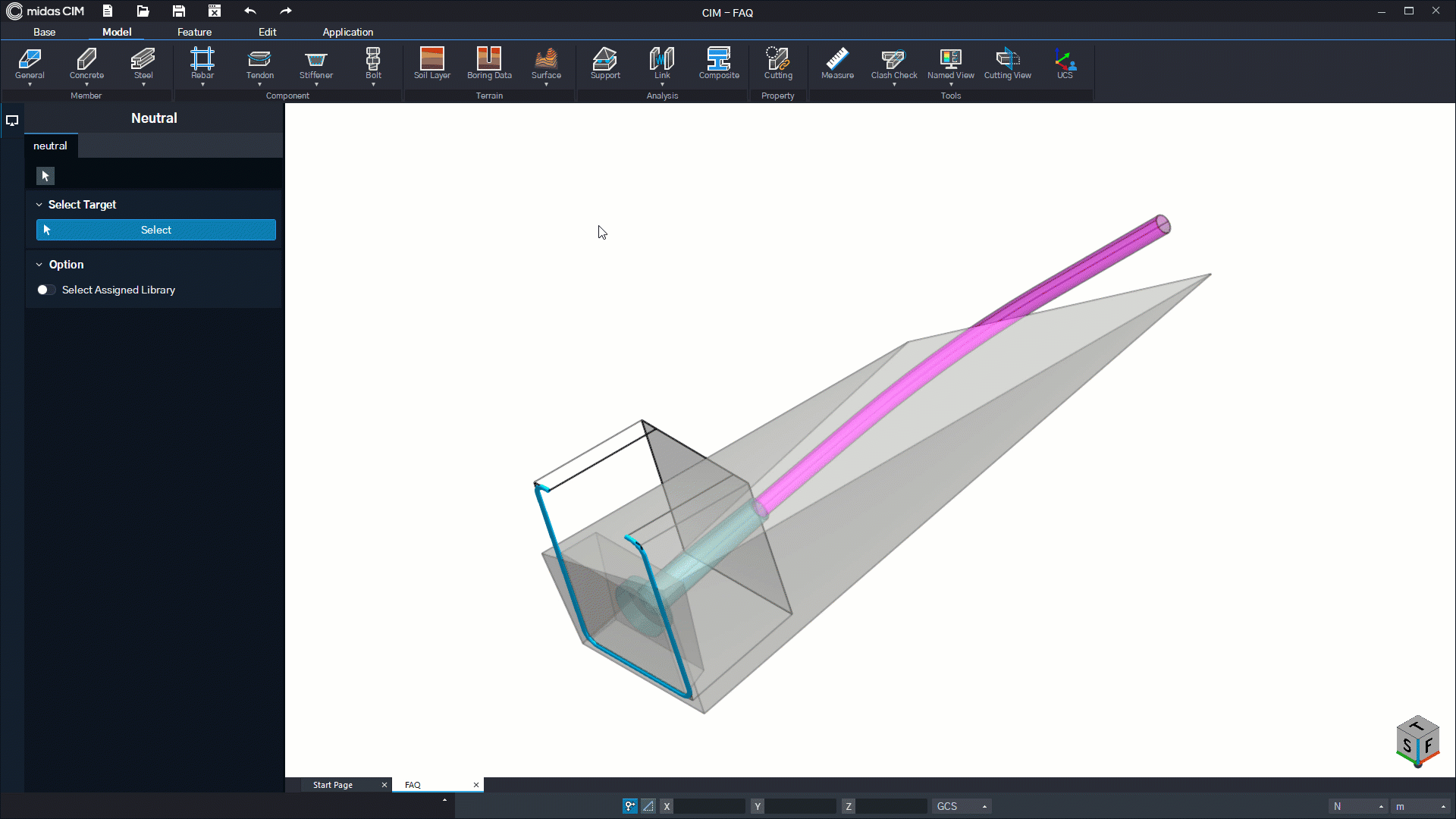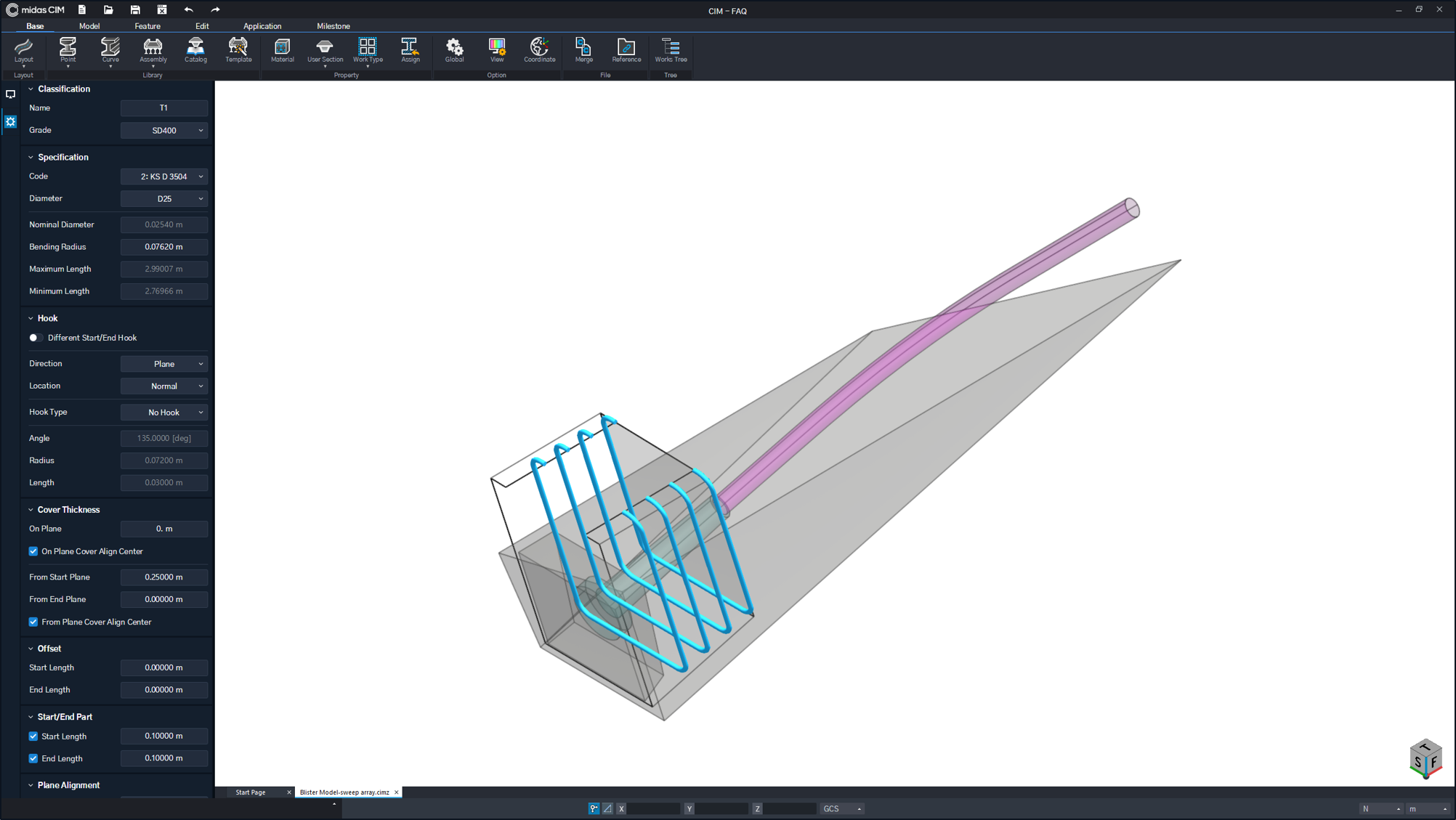Figure. 5 Sweep Array operation(From Start plane Cover thickness_0.25m) Figure. 6 Modeling result through Sweep Array

Figure 5 shows modeling using the sweep array function, one of the types of rebar array.

This function is a completely custom method by using the Draw Point information possessed when the rebar is drawn to designate the direction of each point between the arrays as an arbitrary path.

Paths that can be immediately extracted from the model without CAD-based lines can be used, so it is a function with very high accuracy and freedom.

Sweep Array is a function to create a rebar dependent on an object, but since the shape of the rebar is directly customized, there is a limitation that it does not respond immediately to the shape change of the object.

Case 3.
Modeling using Void Member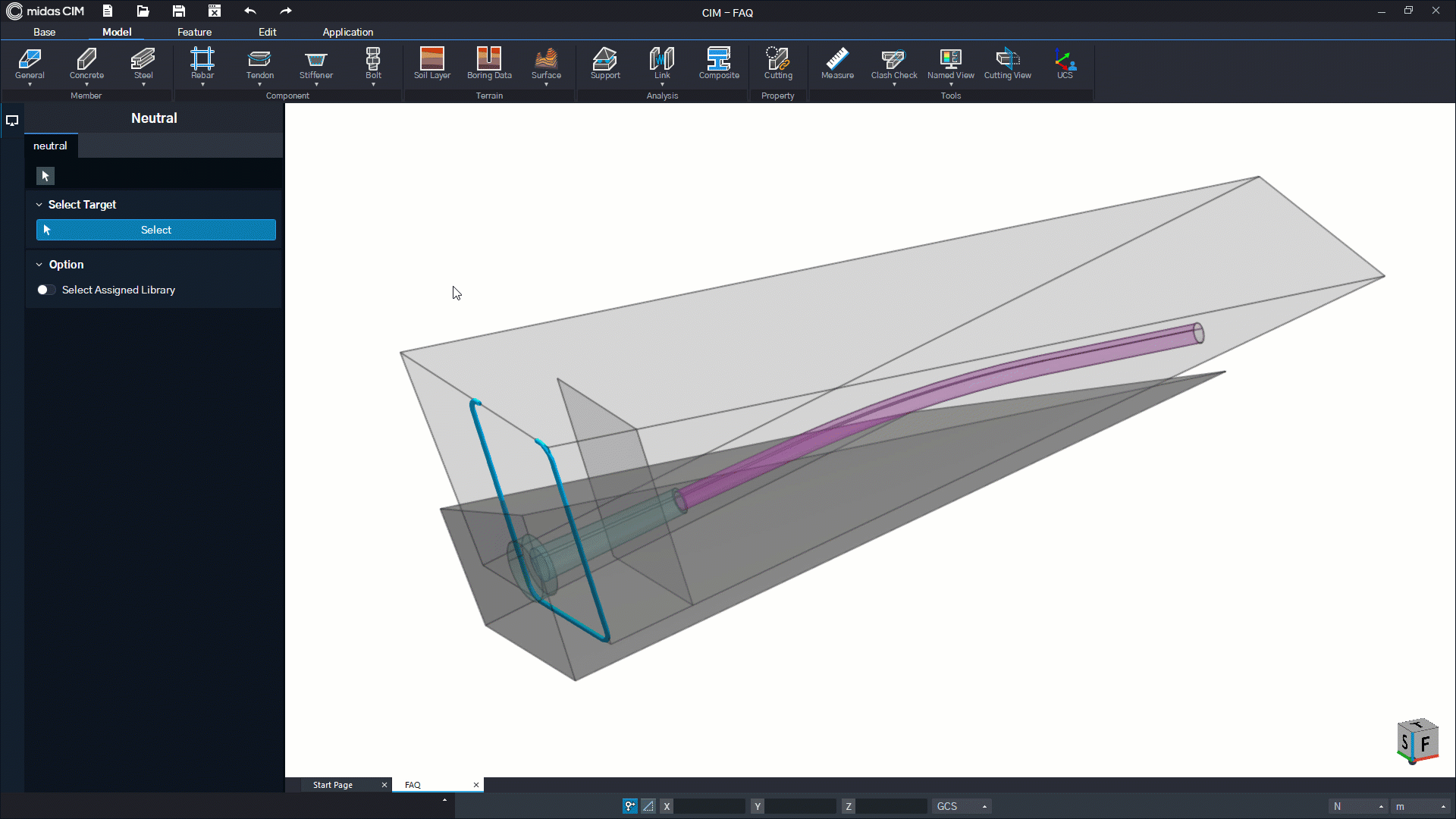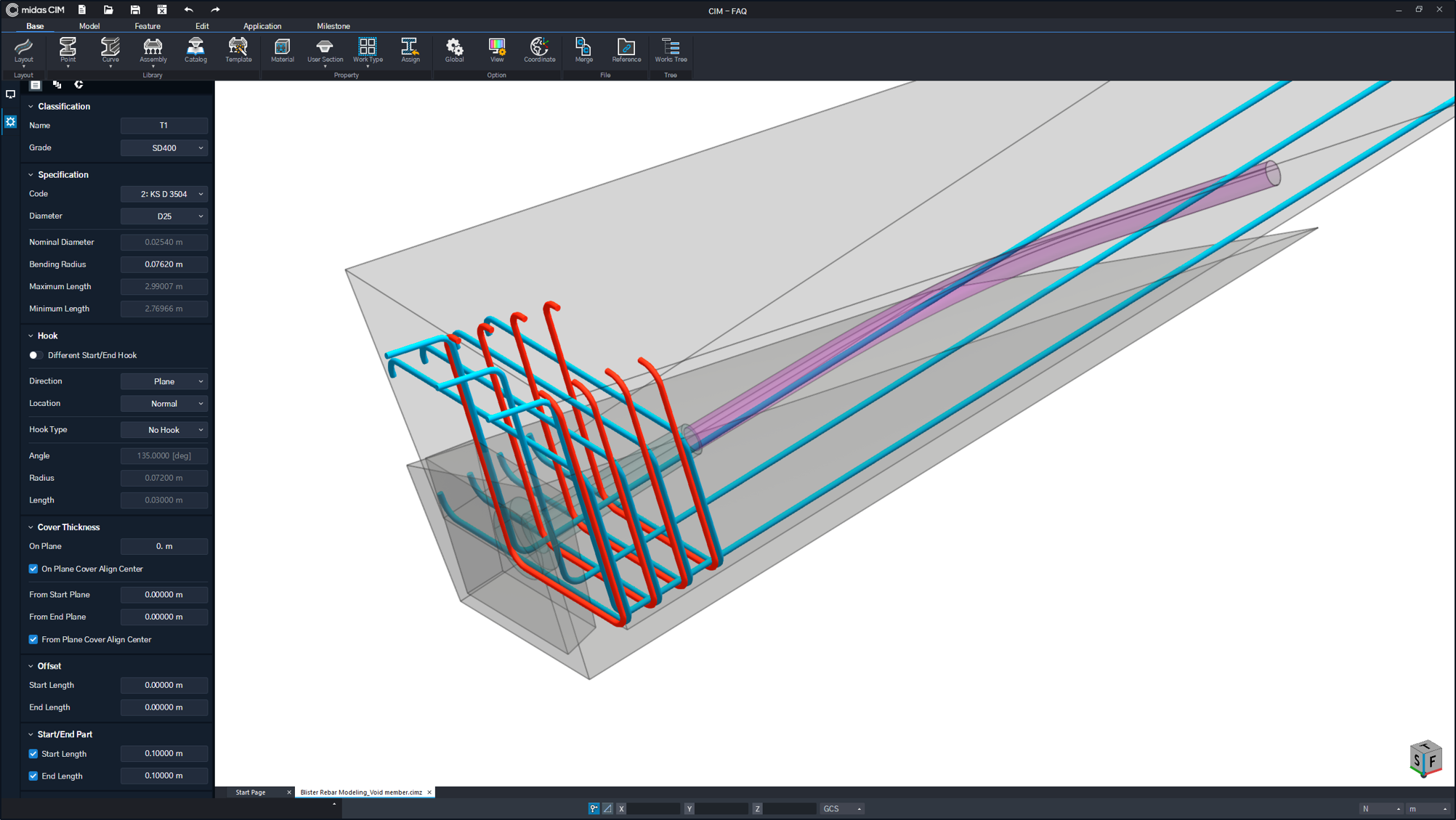Figure. 7 Tapered Array using Void object Figure. 8 Modeling result using Void object

This is a Rebar Modeling method using an object with Member Type set to Void.

A void object can be set by changing the type of concrete member, but it is a dummy member that does not have any effect on analysis and quantity calculation, and exists in a semi-transparent state graphically.

Array in the above method is the same as the general CIM rebar modeling procedure, so if you change the shape of the Void object,
The advantage is that the rebar responds automatically and has all shape-related properties.

However, it is necessary to additionally create a dummy member for rebar modeling, and the difficulty of work may be determined according to the user's skill level.

Rebar modeling of various atypical structures can be carried out using the methods introduced above. Consider the pros and cons of each function and proceed with Rebar Modeling appropriate to the situation.

0